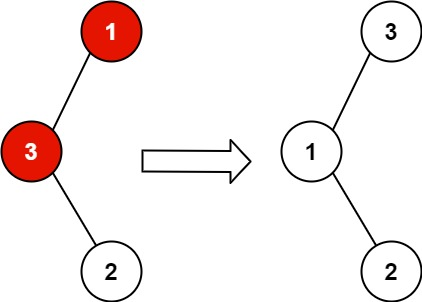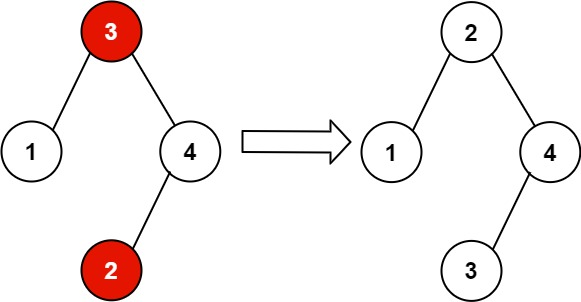## Algorithm

Problem Nmae: 99. Recover Binary Search Tree

You are given the `root` of a binary search tree (BST), where the values of exactly two nodes of the tree were swapped by mistake. Recover the tree without changing its structure.

Example 1:```Input: root = [1,3,null,null,2]
Output: [3,1,null,null,2]
Explanation: 3 cannot be a left child of 1 because 3 > 1. Swapping 1 and 3 makes the BST valid.
```

Example 2:```Input: root = [3,1,4,null,null,2]
Output: [2,1,4,null,null,3]
Explanation: 2 cannot be in the right subtree of 3 because 2 < 3. Swapping 2 and 3 makes the BST valid.
```

Constraints:

• The number of nodes in the tree is in the range `[2, 1000]`.
• `-231 <= Node.val <= 231 - 1`

## Code Examples

### #1 Code Example with C Programming

```Code - C Programming```

``````
void find_nodes(struct TreeNode *node,
struct TreeNode **n1,
struct TreeNode **n2,
struct TreeNode **n3,
struct TreeNode **p) {
if (!node || *n3) return;            // all are found

find_nodes(node->left, n1, n2, n3, p);

if (*p && (*p)->val > node->val) {  // found two nodes in wrong order
if (!*n1) {
*n1 = *p;                   // save first node
*n2 = node;                 // save second node
} else {
*n3 = node;                 // save third node
return;                     // all are found
}
}
*p = node;

find_nodes(node->right, n1, n2, n3, p);
}
void recoverTree(struct TreeNode* root) {
struct TreeNode *n1, *n2, *n3, *p;
int k;

n1 = n2 = n3 = p = NULL;

find_nodes(root, &n1, &n2, &n3, &p);

if (n1) {
if (!n3) n3 = n2;
k = n1->val;            // swap first and third node
n1->val = n3->val;
n3->val = k;
}
}
``````
Copy The Code &

Input

cmd
root = [1,3,null,null,2]

Output

cmd
[3,1,null,null,2]

### #2 Code Example with C++ Programming

```Code - C++ Programming```

``````
class Solution {
public:
void recoverTree(TreeNode* root) {
TreeNode* pre = NULL, *one = NULL, *two = NULL;
DFS(root, pre, one, two);
swap(one->val, two->val);
}

void DFS(TreeNode* cur, TreeNode* &pre, TreeNode* &one, TreeNode* &two){
if(!cur) return;
DFS(cur->left, pre, one, two);
if(pre && cur->val < pre->val){
if(!one) one = pre;
two = cur;
}
pre = cur;
DFS(cur->right, pre, one, two);
}
};
``````
Copy The Code &

Input

cmd
root = [1,3,null,null,2]

Output

cmd
[3,1,null,null,2]

### #3 Code Example with Java Programming

```Code - Java Programming```

``````
class Solution {
public void recoverTree(TreeNode root) {
TreeNode nodeOne = null;
TreeNode nodeTwo = null;
TreeNode prevNode = null;
Stack stack = new Stack<>();
while (root != null || !stack.isEmpty()) {
while (root != null) {
stack.push(root);
root = root.left;
}
if (!stack.isEmpty()) {
root = stack.pop();
if (nodeOne == null && prevNode != null && prevNode.val > root.val) {
nodeOne = prevNode;
}
if (nodeOne != null && prevNode.val > root.val) {
nodeTwo = root;
}
prevNode = root;
root = root.right;
}
}
int tempValue = nodeOne.val;
nodeOne.val = nodeTwo.val;
nodeTwo.val = tempValue;
}
}
``````
Copy The Code &

Input

cmd
root = [3,1,4,null,null,2]

### #4 Code Example with Javascript Programming

```Code - Javascript Programming```

``````
const recoverTree = function(root) {
let node1, node2;
let prev = new TreeNode(-Infinity);
traverse(root);
swap(node1, node2)

function traverse(node) {
if (!node) return;
traverse(node.left);
if (node.val < prev.val) {
node2 = node;
if (!node1) node1 = prev;
}
prev = node;
traverse(node.right);
}

function swap(node1, node2) {
let temp = node1.val
node1.val = node2.val
node2.val = temp
}
}
``````
Copy The Code &

Input

cmd
root = [3,1,4,null,null,2]

Output

cmd
[2,1,4,null,null,3]

### #5 Code Example with Python Programming

```Code - Python Programming```

``````
class Solution:
def recoverTree(self, root):
def inorder(node):
if node.left:
yield from inorder(node.left)
yield node
if node.right:
yield from inorder(node.right)
swap1 = swap2 = smaller = None
for node in inorder(root):
if smaller and smaller.val > node.val:
if not swap1:
swap1 = smaller
swap2 = node
smaller = node
if swap1:
swap1.val, swap2.val = swap2.val, swap1.val
``````
Copy The Code &

Input

cmd
root = [1,3,null,null,2]

Output

cmd
[3,1,null,null,2]

### #6 Code Example with C# Programming

```Code - C# Programming```

``````
using System.Collections.Generic;

namespace LeetCode
{
public class _099_RecoverBinarySearchTree
{
private Stack stack = new Stack();
private TreeNode prev = null;
private TreeNode first = null;
private TreeNode second = null;
private TreeNode current = null;

public void RecoverTree(TreeNode root)
{
current = root;
while (stack.Count > 0 || current != null)
{
while (current != null)
{
stack.Push(current);
current = current.left;
}

current = stack.Pop();
if (prev != null && current.val < prev.val)
{
if (first == null) first = prev;
second = current;
}
if (first != null && first.val < current.val) break;

prev = current;
current = current.right;
}

var temp = first.val;
first.val = second.val;
second.val = temp;
}
}
}
``````
Copy The Code &

Input

cmd
root = [1,3,null,null,2]

Output

cmd
[3,1,null,null,2]